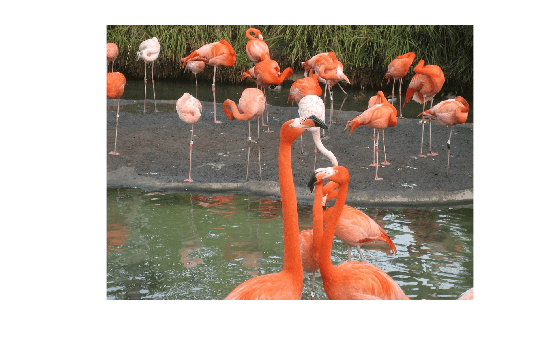# randomWindow2d

Randomly select rectangular region in image

## Syntax

``win = randomWindow2d(inputSize,targetSize)``
``win = randomWindow2d(inputSize,'Scale',scale,'DimensionRatio',dimensionRatio)``

## Description

example

````win = randomWindow2d(inputSize,targetSize)` selects a rectangular region of size `targetSize` from a random position in an image of size `inputSize`.```

example

````win = randomWindow2d(inputSize,'Scale',scale,'DimensionRatio',dimensionRatio)` selects a rectangular region, specifying the size of the region relative to the input image, `scale`, and the aspect ratio of the region, `dimensionRatio`.```

## Examples

collapse all

```I = imread("flamingos.jpg"); imshow(I)```Specify the size of the input image and the target size of the rectangular region.

```inputSize = size(I); targetSize = [40 60];```

Select a region of the target size from a random location in the image.

`rect = randomWindow2d(inputSize,targetSize);`

Convert the region from a `Rectangle` object to a 4-element vector of the form [xmin ymin width height].

```rectXYWH = [rect.XLimits(1) rect.YLimits(1) ... diff(rect.XLimits)+1 diff(rect.YLimits)+1];```

Display the boundary of the rectangular region overlaid on the original image.

```annotatedI = insertShape(I,"rectangle",rectXYWH,"LineWidth",3); imshow(annotatedI)``````I = imread("strawberries.jpg"); imshow(I)```Specify the size of the input image.

`inputSize = size(I);`

Specify a fractional area of the region between 2% and 13% of the area of the input image. Specify a range of aspect ratios between 1:5 and 4:3.

```scale = [0.02 0.13]; dimensionRatio = [1 5;4 3];```

Specify a region with a randomly selected fractional area and aspect ratio from a random location in the image.

`rect = randomWindow2d(inputSize,"Scale",scale,"DimensionRatio",dimensionRatio);`

Crop the original image to the randomly selected region and display the result.

```Icrop = imcrop(I,rect); imshow(Icrop)```## Input Arguments

collapse all

Input image size, specified as one of the following.

Type of Input ImageFormat of `inputSize`
2-D grayscale or binary image2-element vector of positive integers of the form ```[height width]```
2-D RGB or multispectral image 3-element vector of positive integers of the form ```[height width channels]```

Target image size, specified as one of the following.

Type of Target ImageFormat of `targetSize`
2-D grayscale or binary image2-element vector of positive integers of the form ```[height width]```
2-D RGB or multispectral image 3-element vector of positive integers of the form ```[height width channels]```

Region area as a fraction of the input image area, specified as one of these values.

• 2-element nondecreasing numeric vector with values in the range [0, 1]. The elements define a minimum and maximum fractional area of the region, respectively. `randomWindow2d` selects a random value within the range to use as the fractional region area. To use a fixed region area, specify the same value for both elements.

• Function handle. The function must take no input arguments and return one number in the range [0, 1] specifying a valid fractional region area. For more information about function handles, see Create Function Handle.

Range of aspect ratios of the rectangular region, specified as one of these values.

• 2-by-2 matrix of positive numbers. The first row defines the defines the minimum aspect ratio and the second row defines the maximum aspect ratio. `randomWindow2d` selects a random value within the range to use as the aspect ratio. To use a fixed aspect ratio, specify identical values for the first and second rows.

• Function handle. The function must take no input arguments and return one positive number specifying a valid dimension ratio. For example, a value of `1.2` specifies a 5:4 aspect ratio. For more information about function handles, see Create Function Handle.

Example: `[1 8;1 4]` selects an aspect ratio in the range 1:8 to 1:4

## Output Arguments

collapse all

Rectangular window, returned as a `Rectangle` object.

## Version History

Introduced in R2021a